PWN p. 151 #8 question

In PWN the SAT Math Guide (4th Ed, first printing p. 151), Polynomials chapter question #8, you explain the answer by graphing on calculator. Could you explain the answer to this question without graphing, in particular, why A, C and D are true and why B is false? Many thanks!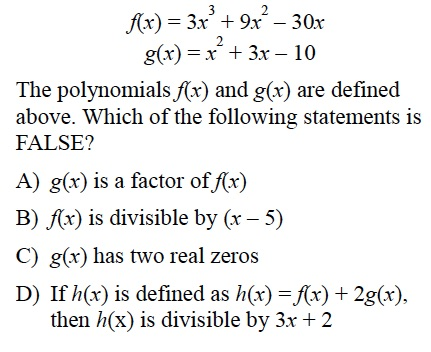Sure! First, recognize that g(x) is factorable: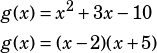From there, you can see that g(x) has two real zeros (2 and –5) so you can eliminate C.

Then, recognize that g(x) is a factor of f(x) as choice A says:Obviously that confirms that choice A is true, so you can eliminate A. However, that also confirms that choice B is false! Remember how we already factored g(x)!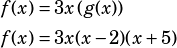f(x) is divisible by (x + 5), but definitely not by (x – 5). You know from the way the question is constructed that only one choice will be false, so once you’ve found it, you can stop working and start bubbling. 🙂

Since you asked, though, we can also show that D is true from what we’ve already figured out using substitution. We already said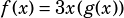, so we can substitute thusly:We can factor a g(x) out of each of those terms!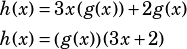Therefore, choice D is also true.